# Lecture 16

## Mergesort

MCS 275 Spring 2022
David Dumas

### Lecture 16: Mergesort

Course bulletins:

• Project 2 due 6pm central Friday, February 25.
• Worksheet 7 will explore the maze solver / generator in more depth.

## Project 2 discussion

You will write functions (mostly recursive) to enumerate integer splittings.

E.g. 1+2+3 and 3+1+2 and 4+2 are splittings of 6

## Plan

• Discuss the theory of
• Divide and conquer
• Sorting
• Mergesort
• Implement mergesort

## Divide and conquer

A strategy that often involves recursion.

• Split a problem into parts.
• Solve for each part.
• Merge the partial solutions into a solution of the original problem.

Not always possible or a good idea. It only works if merging partial solutions is easier than solving the entire problem.

## Comparison sort

Suppose you have a list of objects that can be compared with ==, >, <.

You'd like to reorder them in increasing order.

This problem is called comparison sort. There are many solutions.

## Mergesort

A divide-and-conquer solution to comparison sort.

It is a fast solution, often used in practice.

Key: It is pretty easy to take two sorted lists and merge them into a single sorted list.

So, let's divide our list into halves, sort each one (recursively), then merge them.

Now we'll formalize this.

Algorithm mergesort:

Input: list L whose elements support comparison.

Goal: return a list that contains the items from L but in sorted order.

1. If L has 0 or 1 elements, return L
2. Otherwise, divide L into rougly equal pieces L0 and L1.
3. Use recursive calls to sort L0 and L1.
4. Use merge to merge these sorted lists and return the result.

## Mergesort example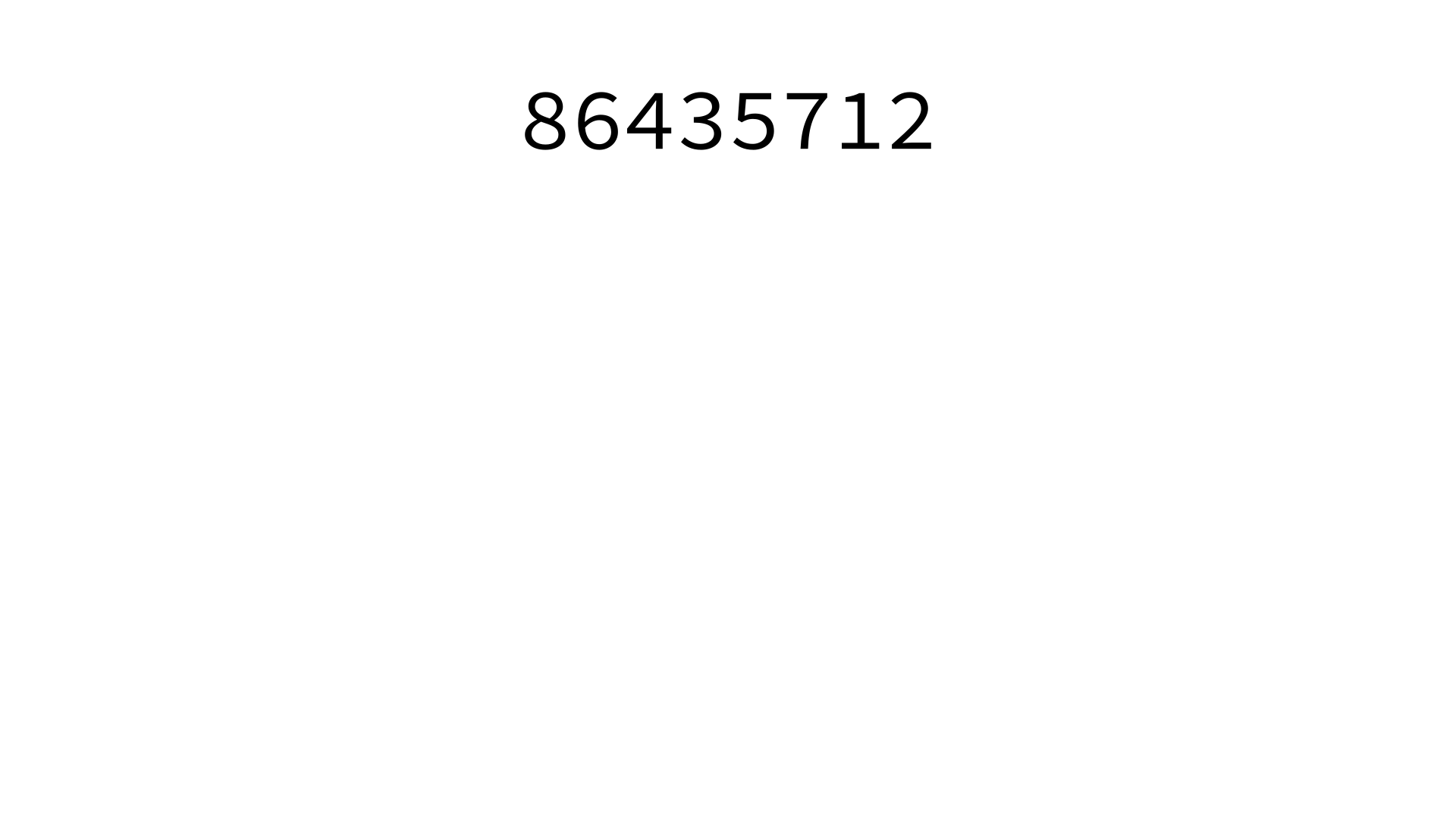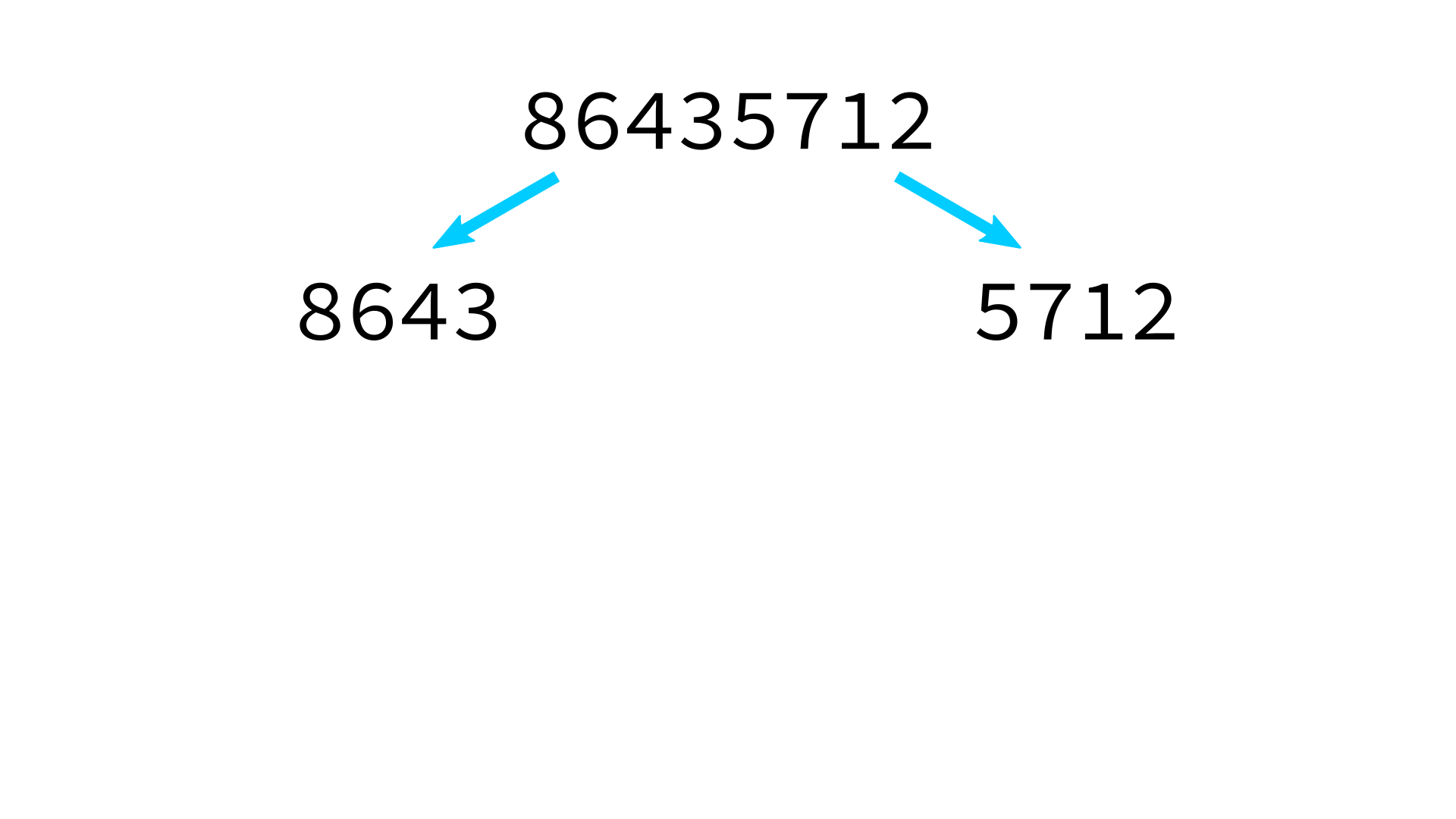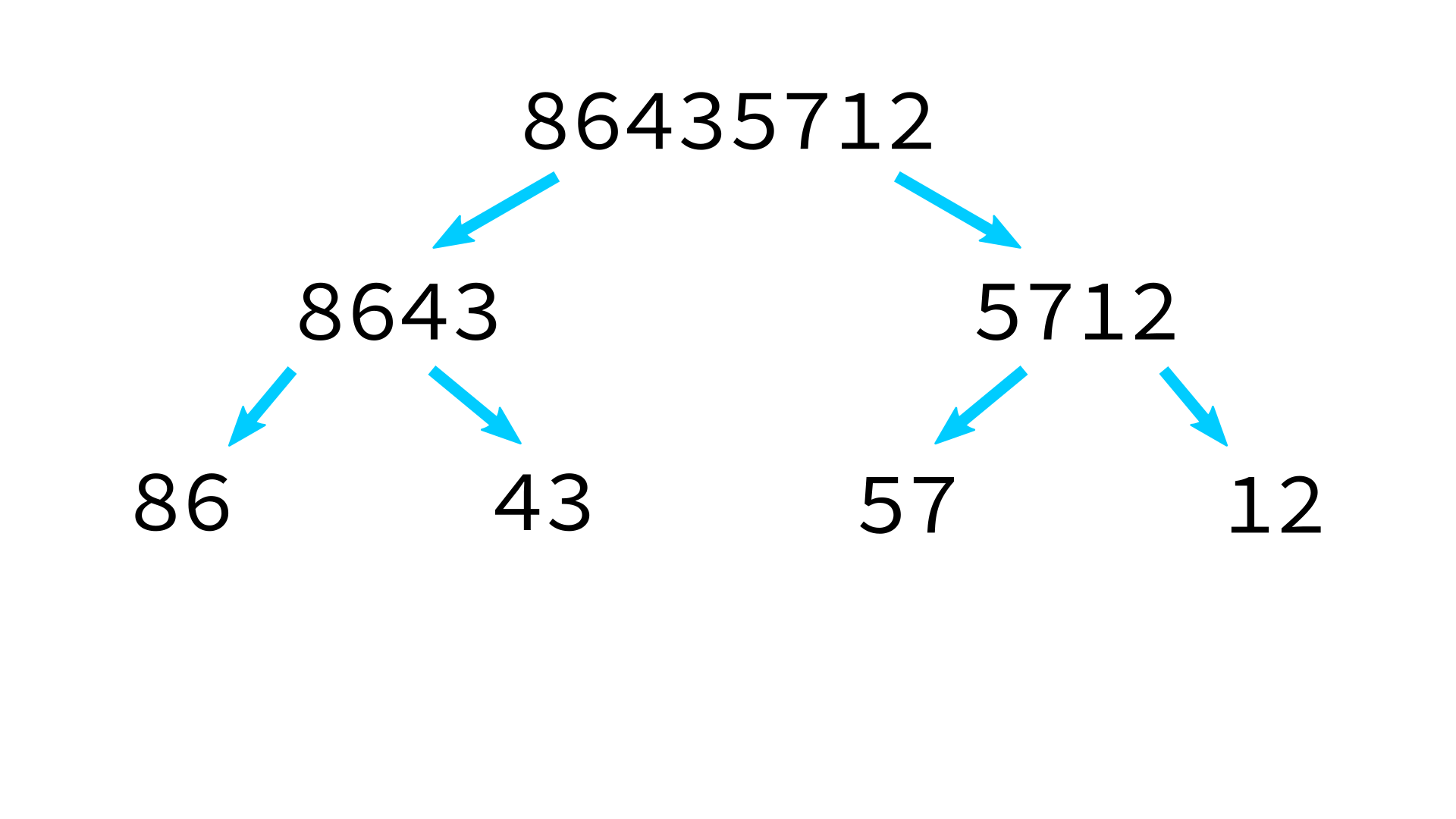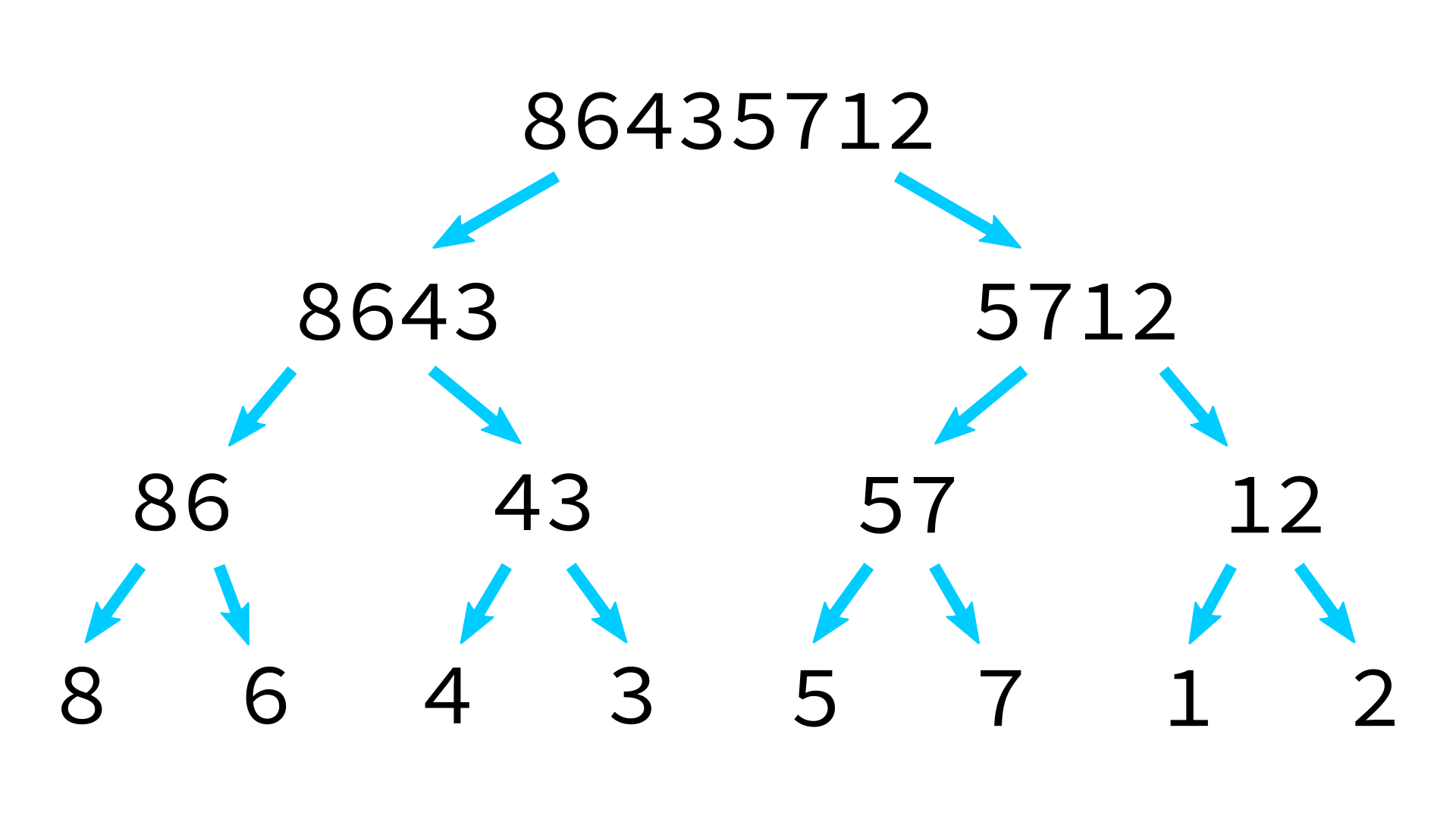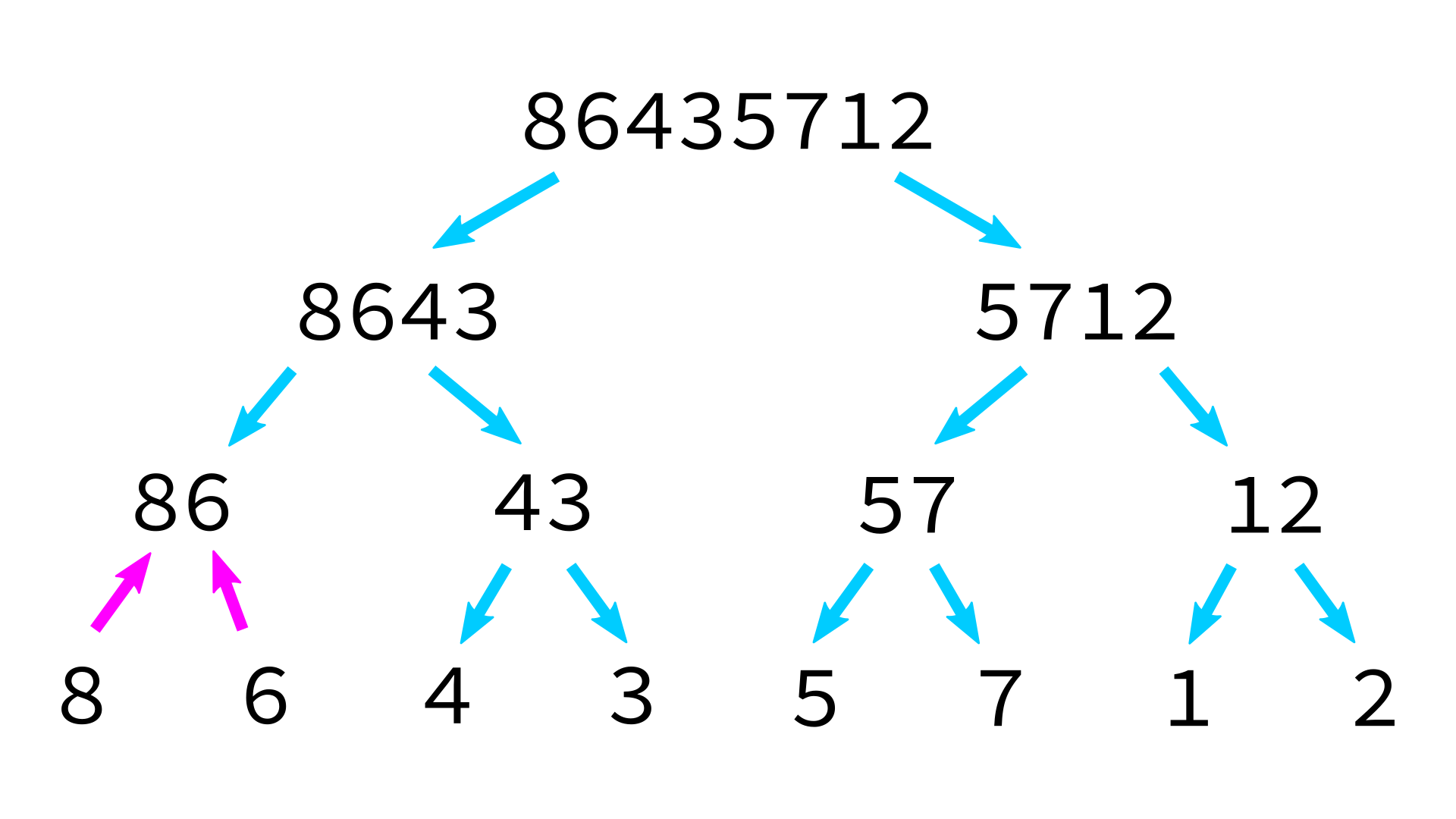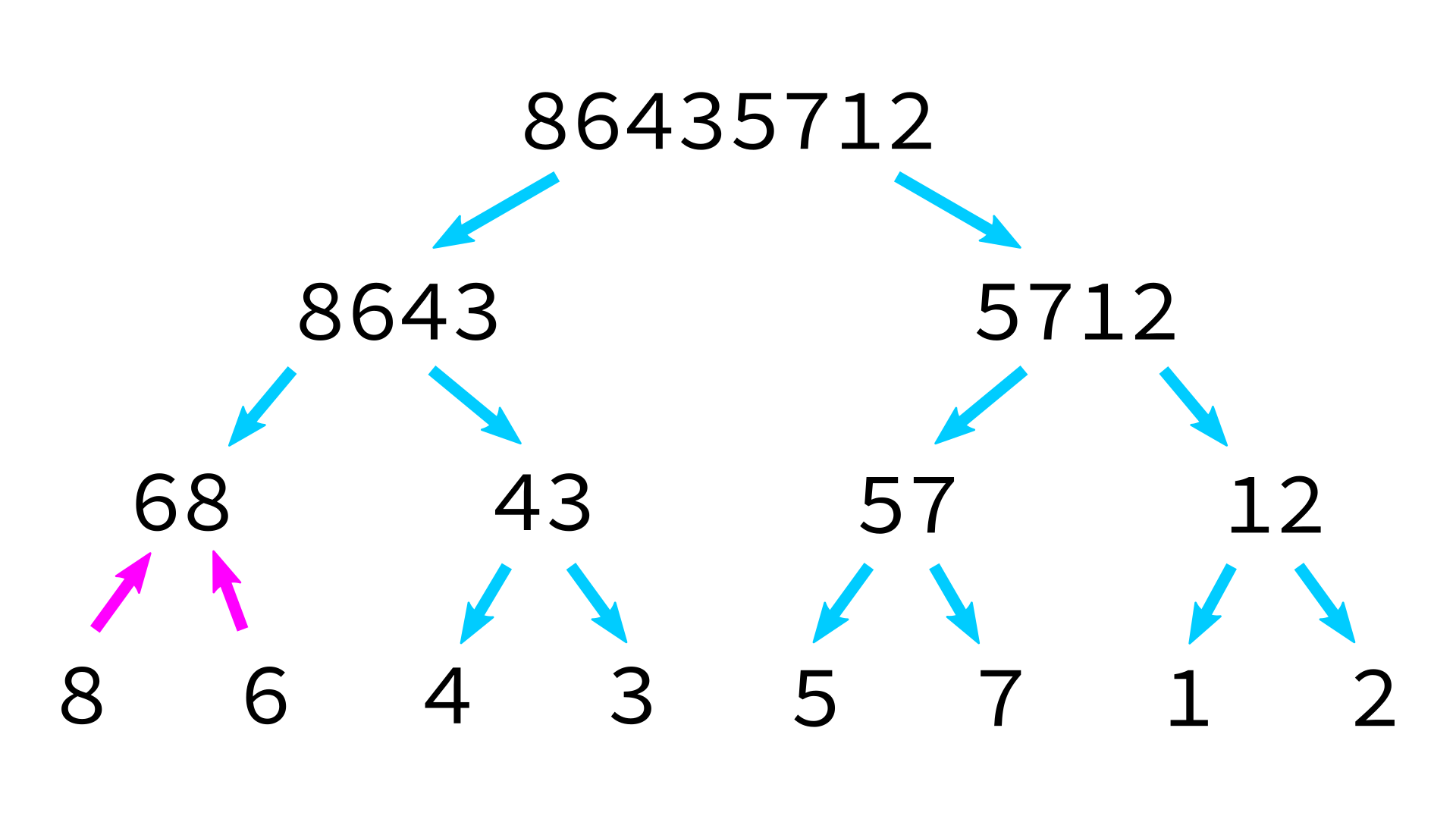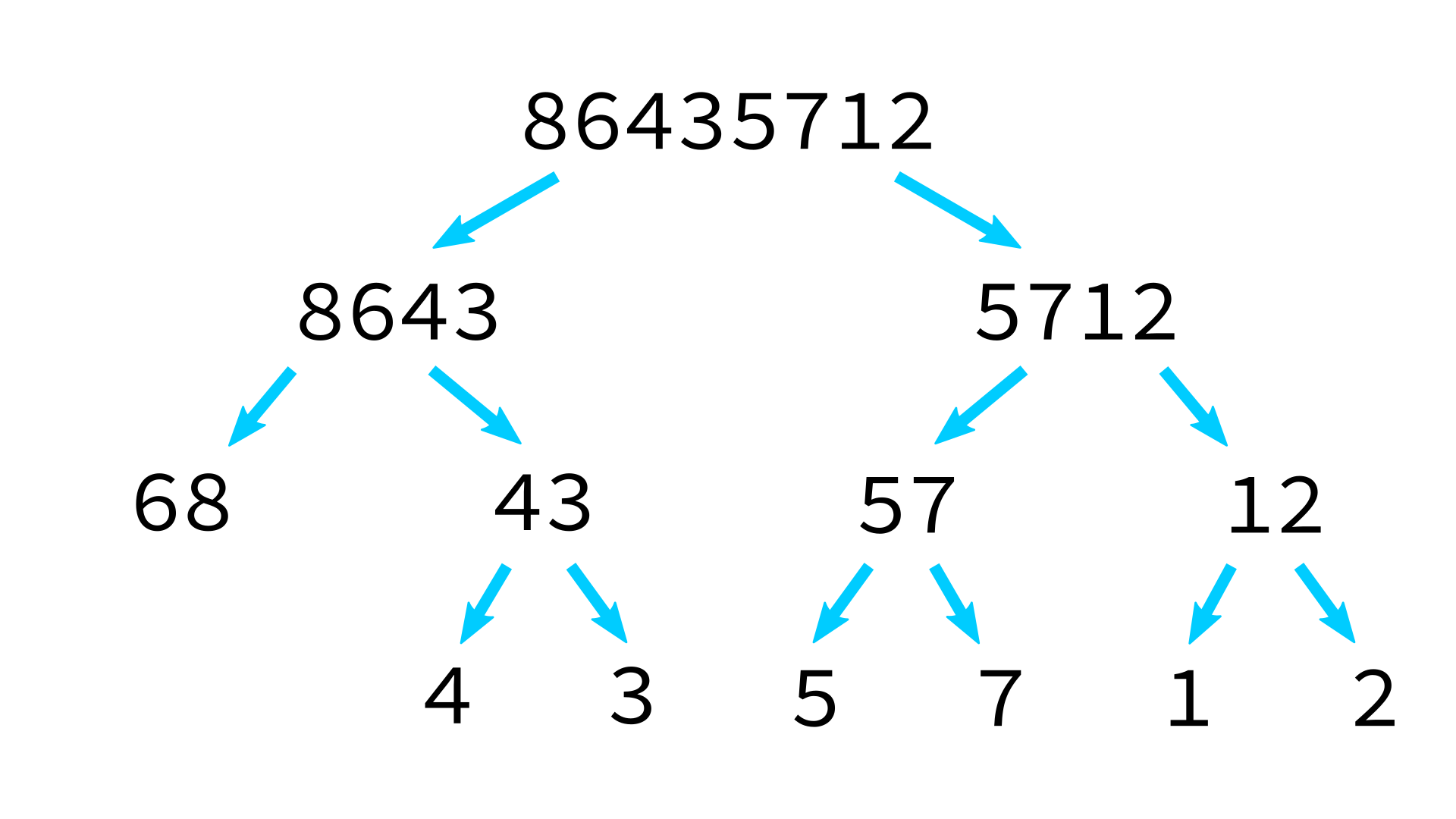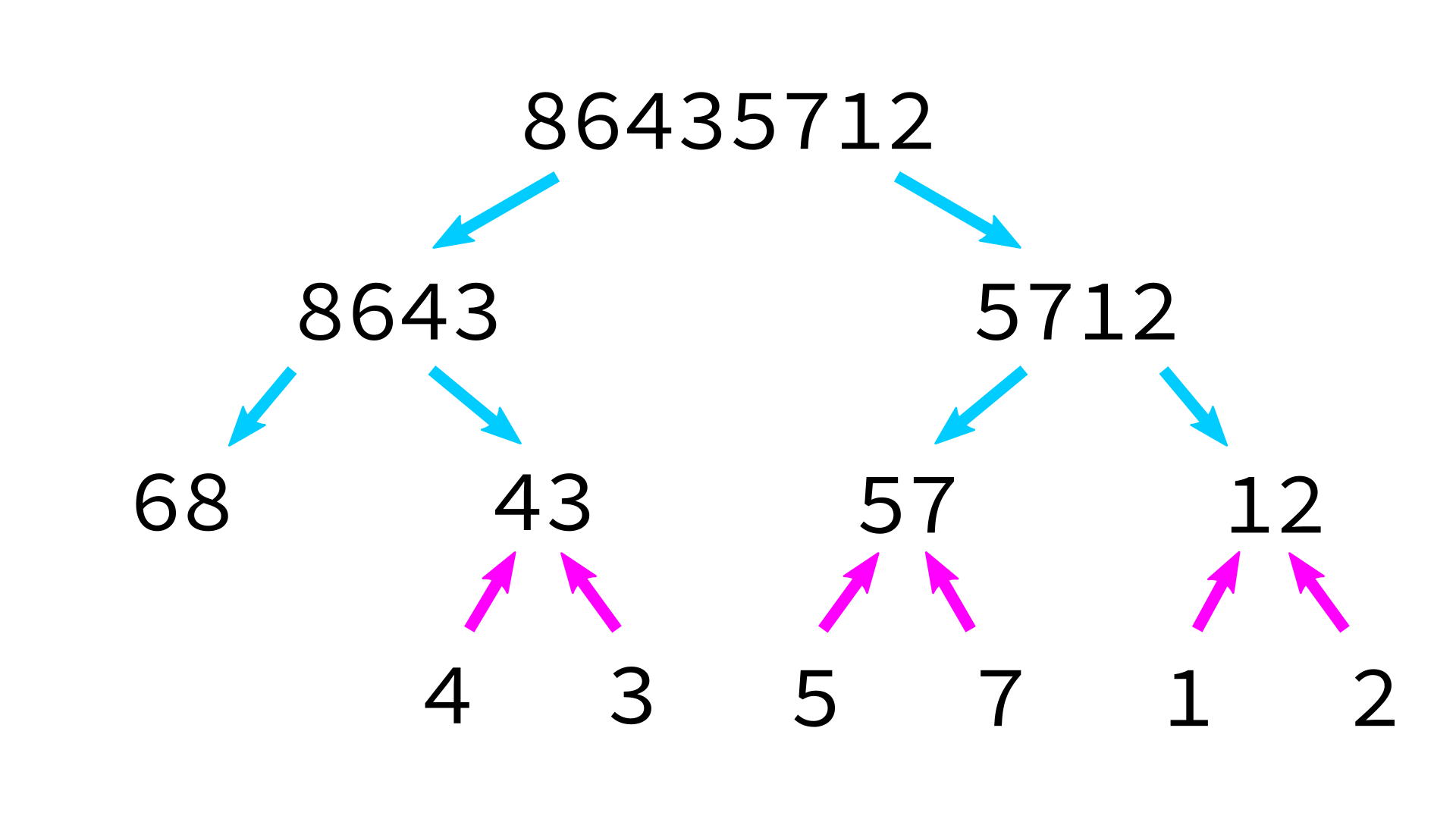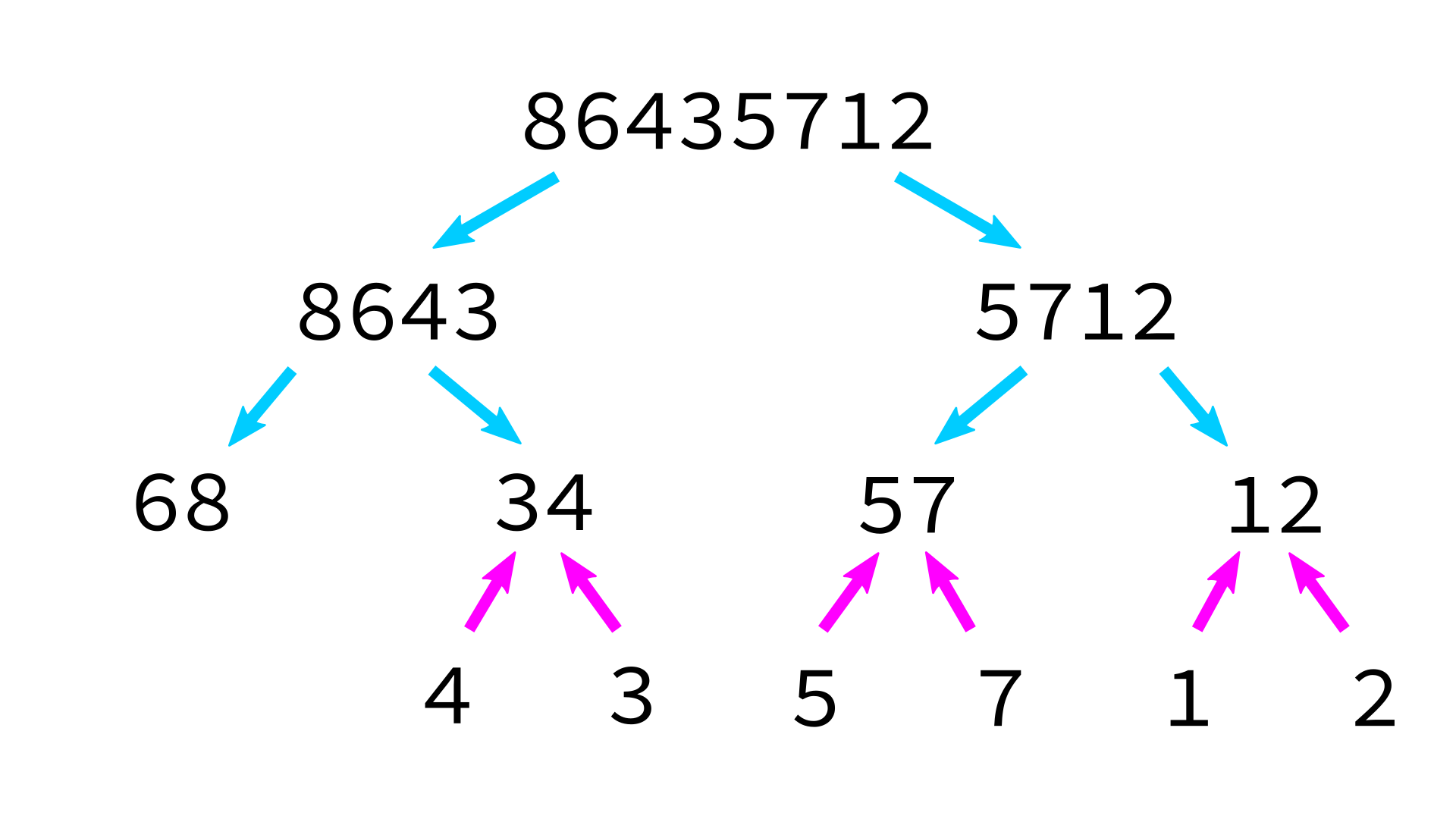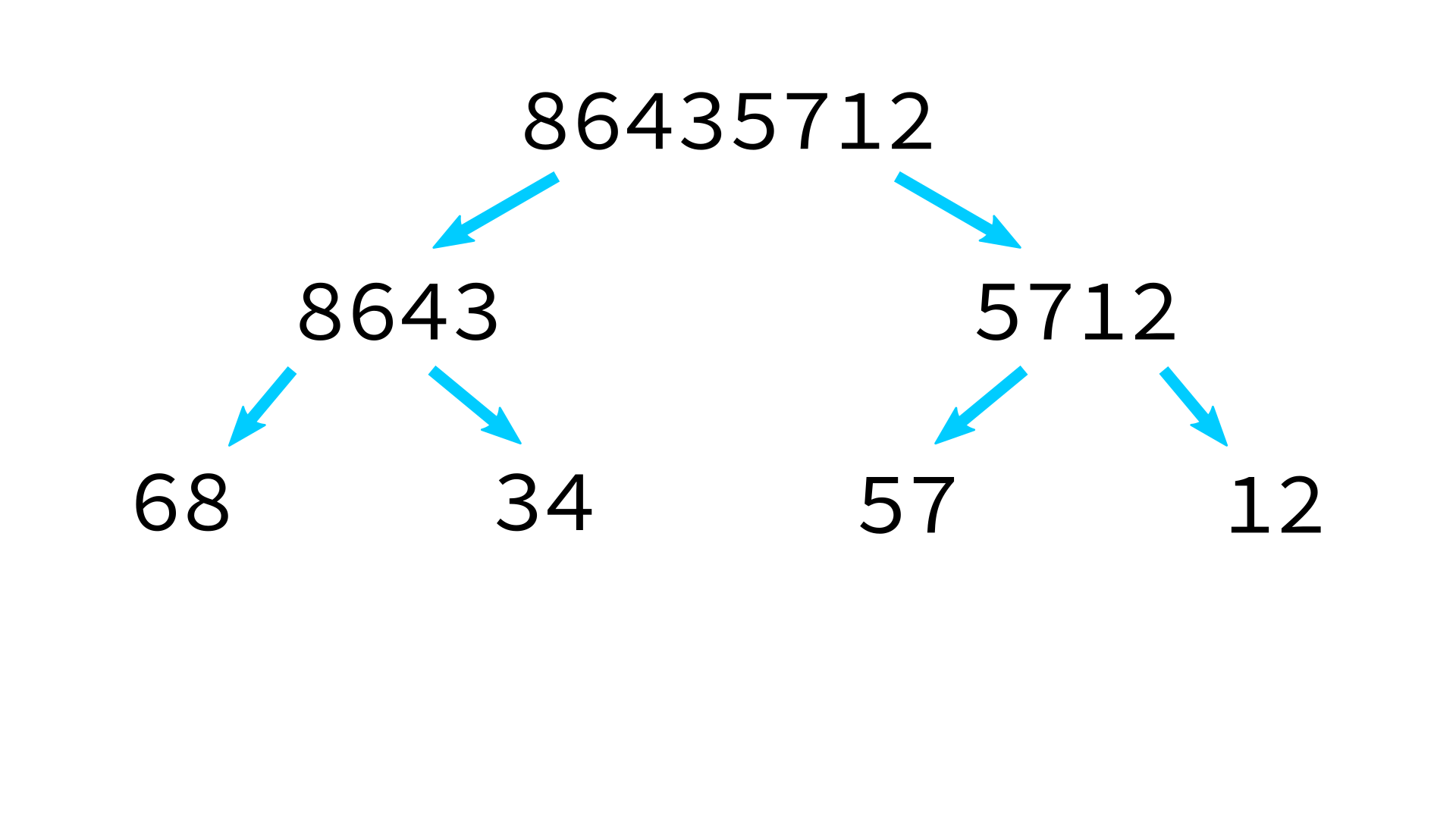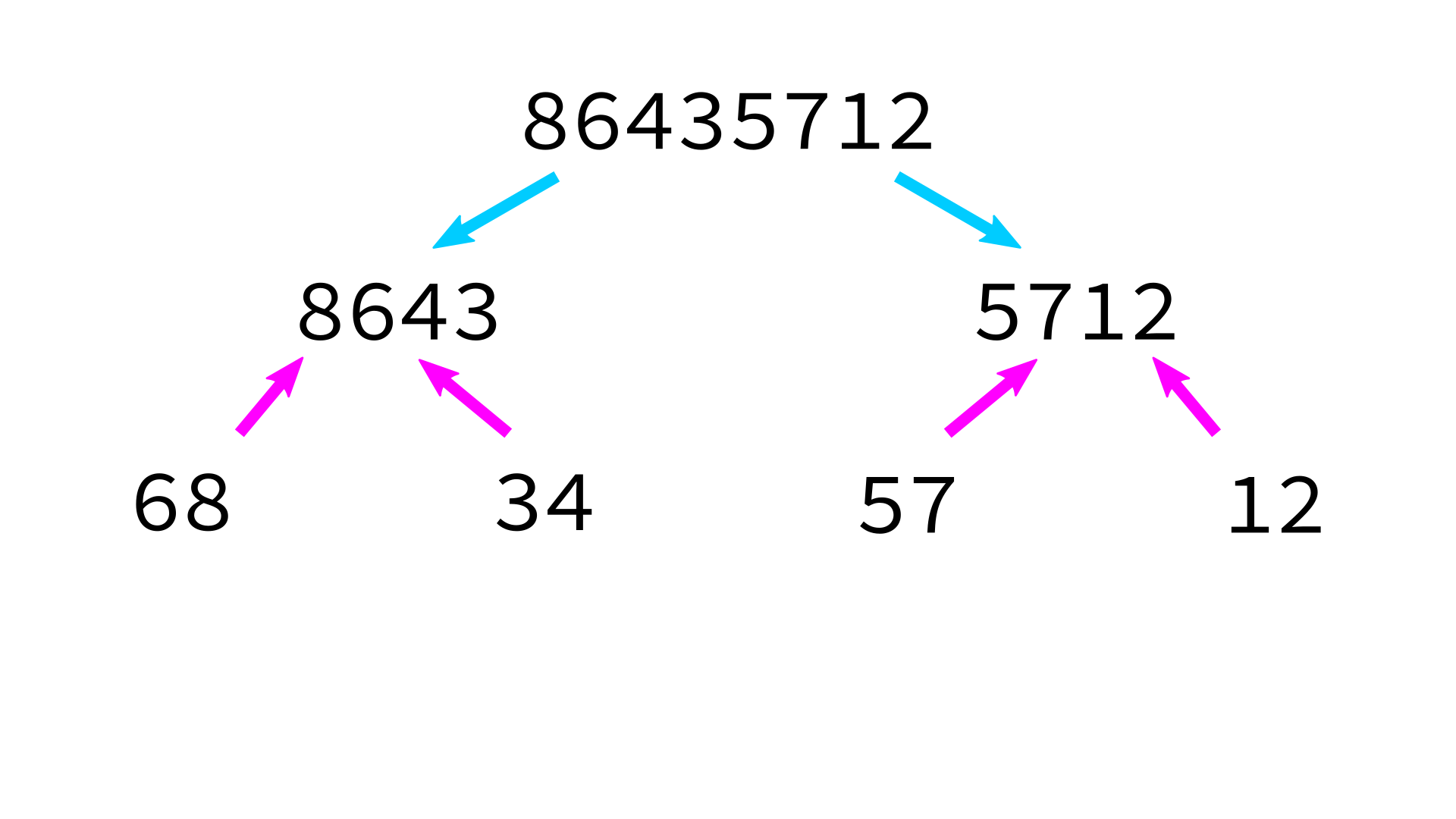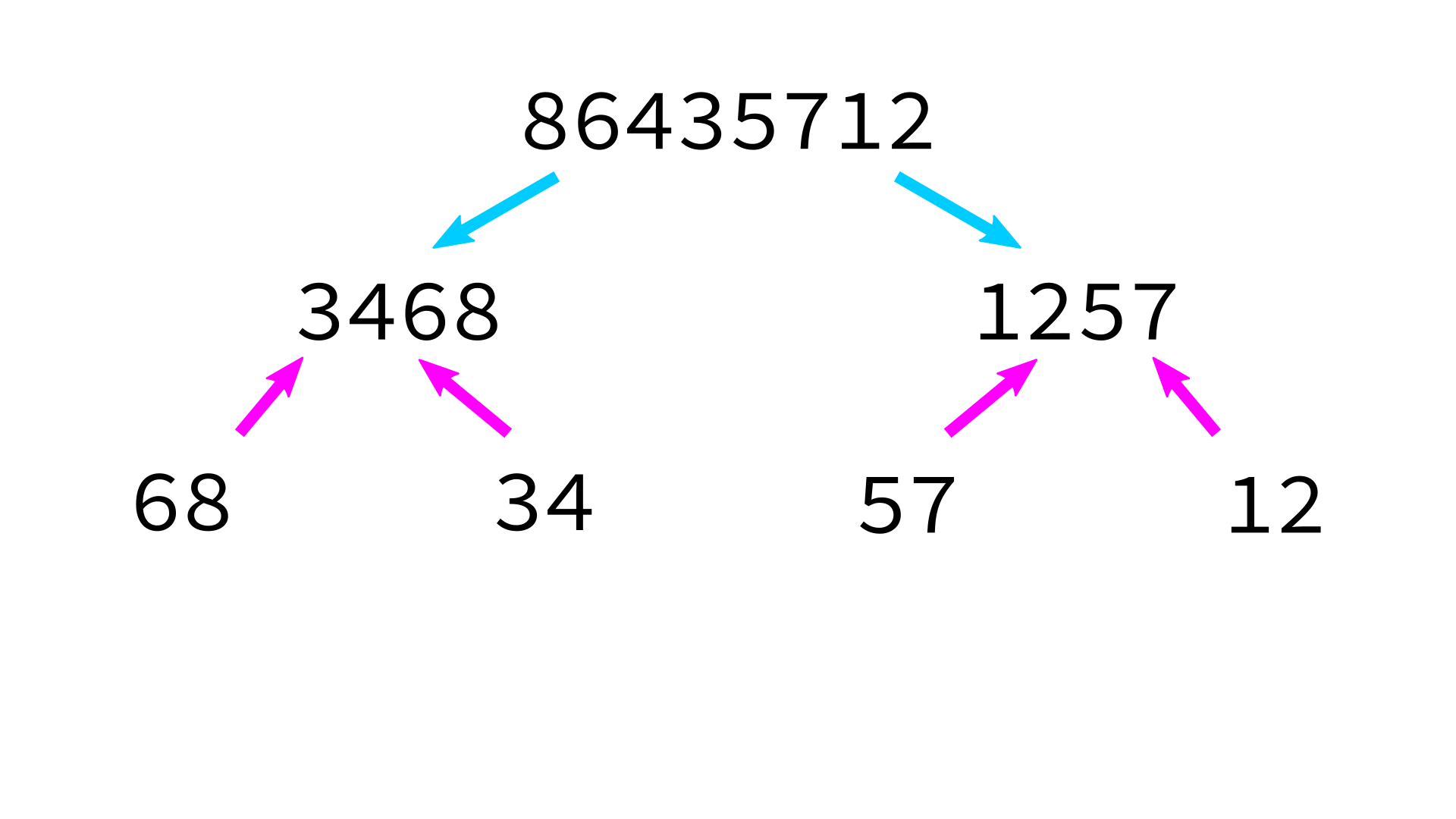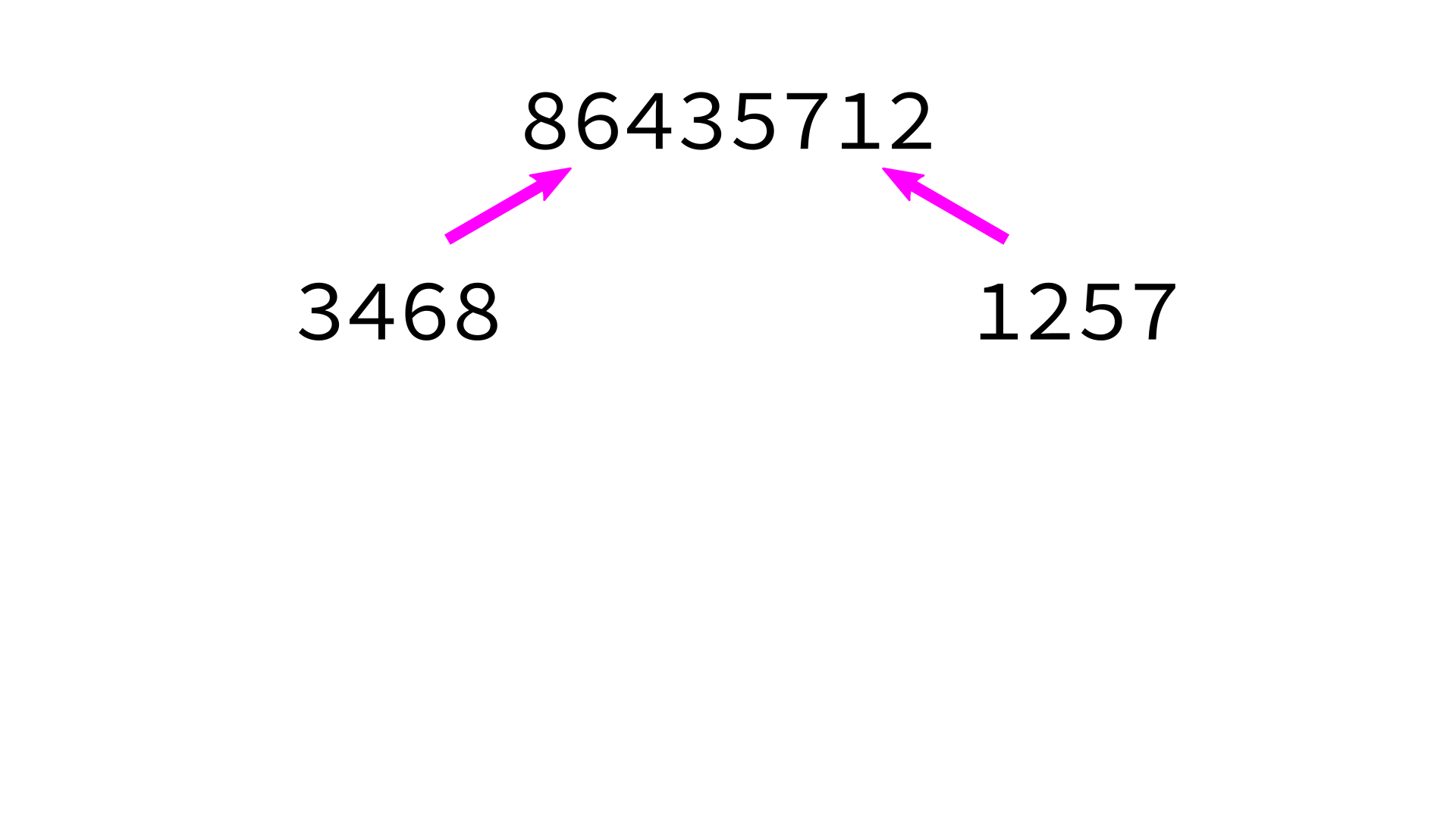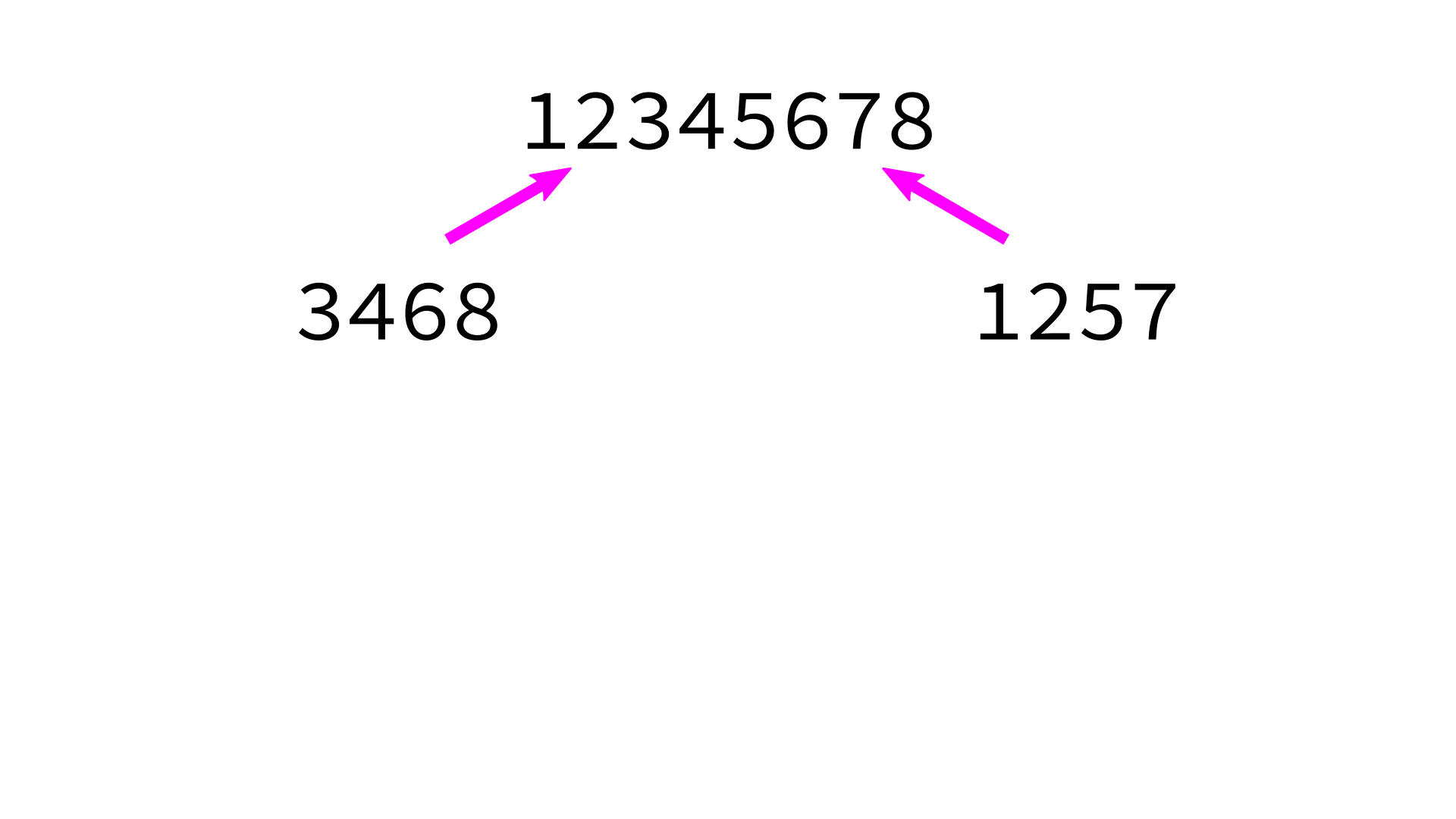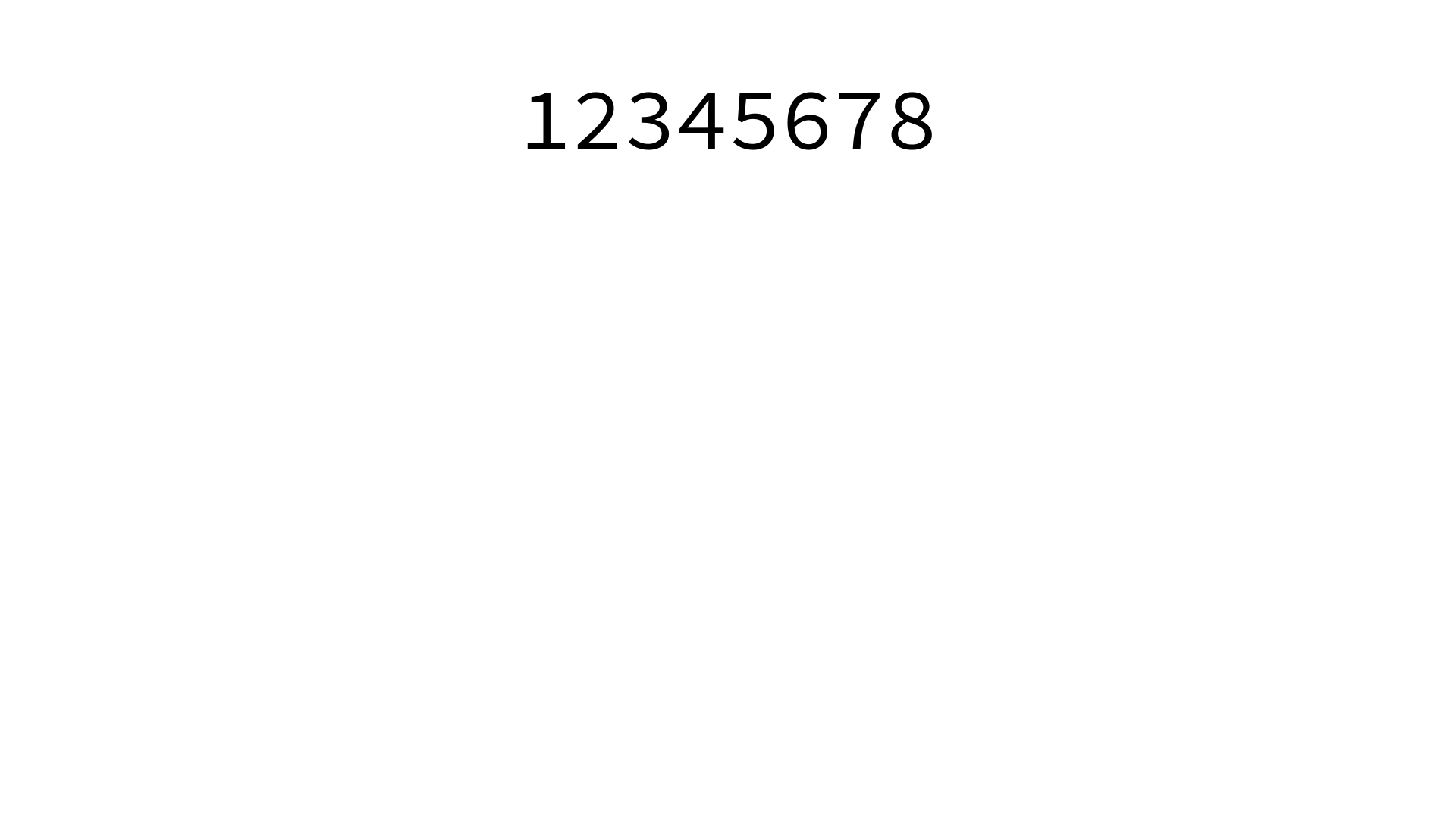## But how to merge?

This algorithm depends on having a function merge that can merge two sorted lists into a single sorted list.

Algorithm merge:

Input: sorted lists L0 and L1.

Goal: return a sorted list with same items as L0+L1

1. Make a new empty list L
2. Make integer variables i0,i1 to keep track of current position in L0,L1 respectively. Set to zero.
3. While i0 < len(L0) and i1 < len(L1), do the following:
• Check which of L0[i0] and L1[i1] is smaller.
• Append the smaller one to L.
• Increment whichever one of i0,i1 was used.
4. Append any remaining portion of L0 to L.
5. Append any remaining portion of L1 to L.

## Merging sorted lists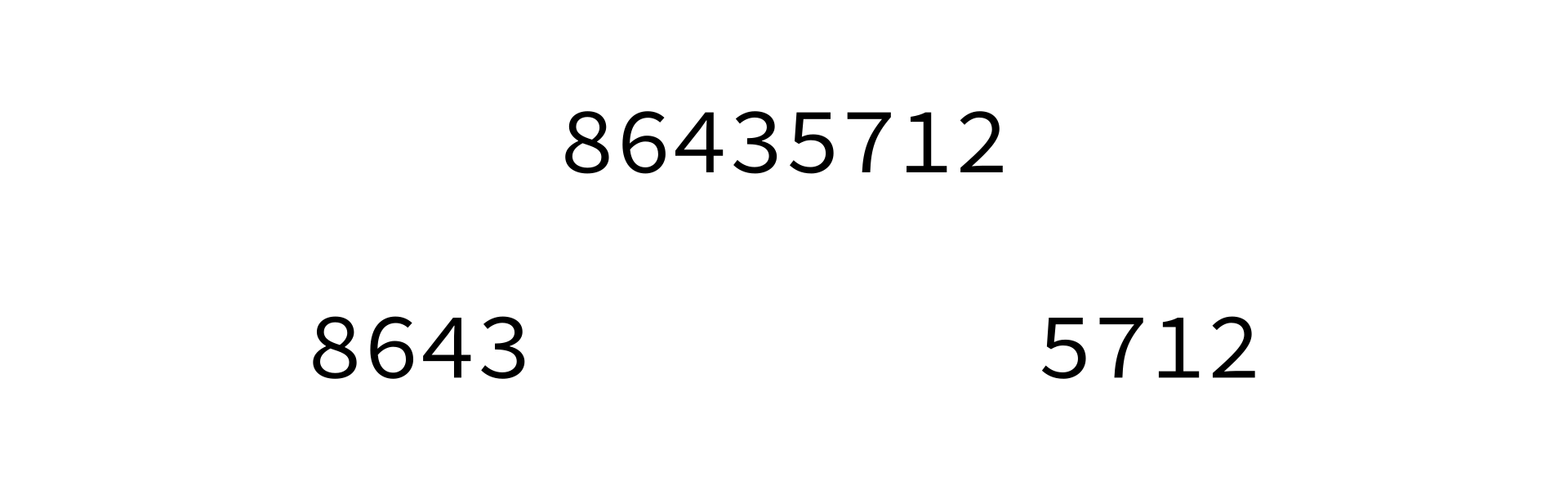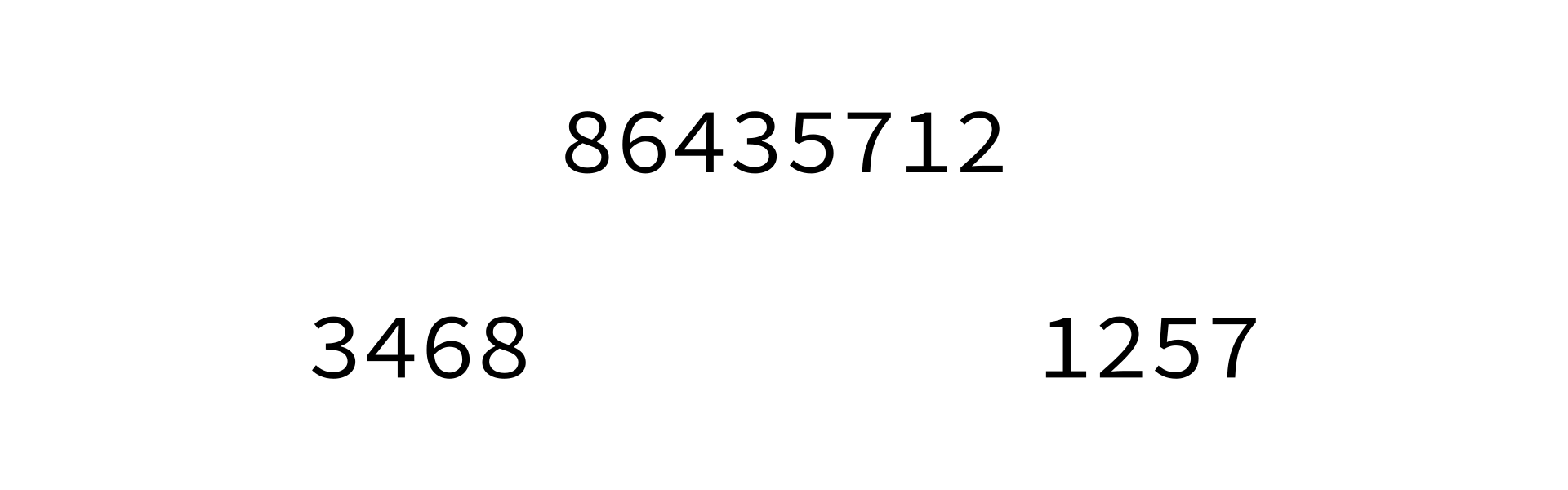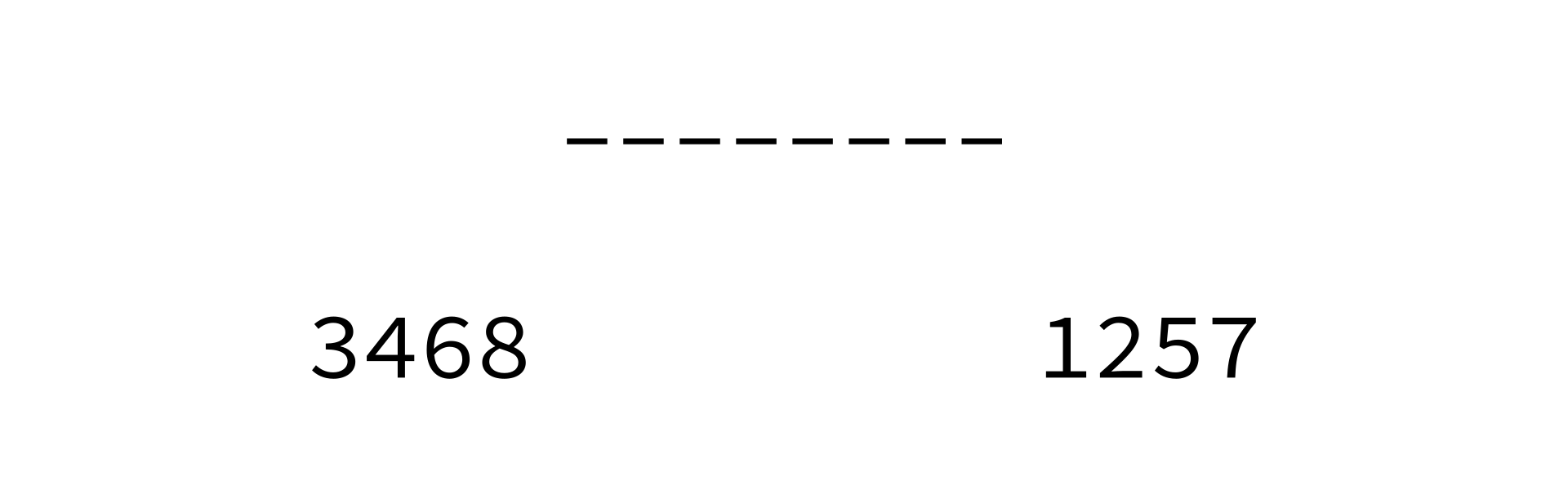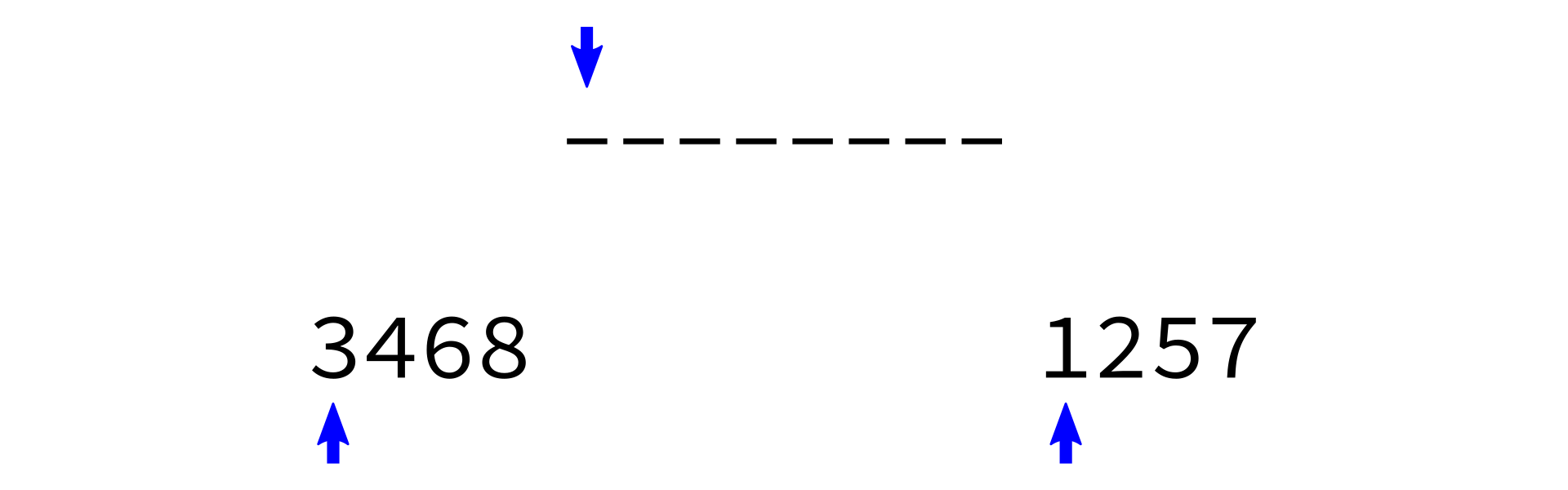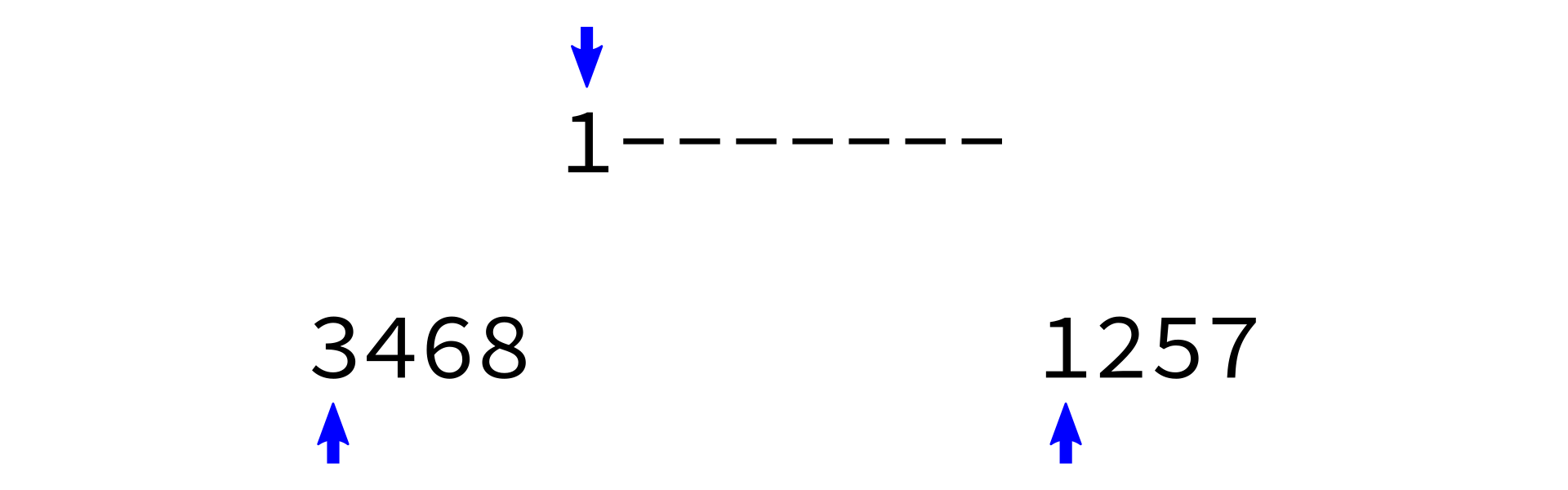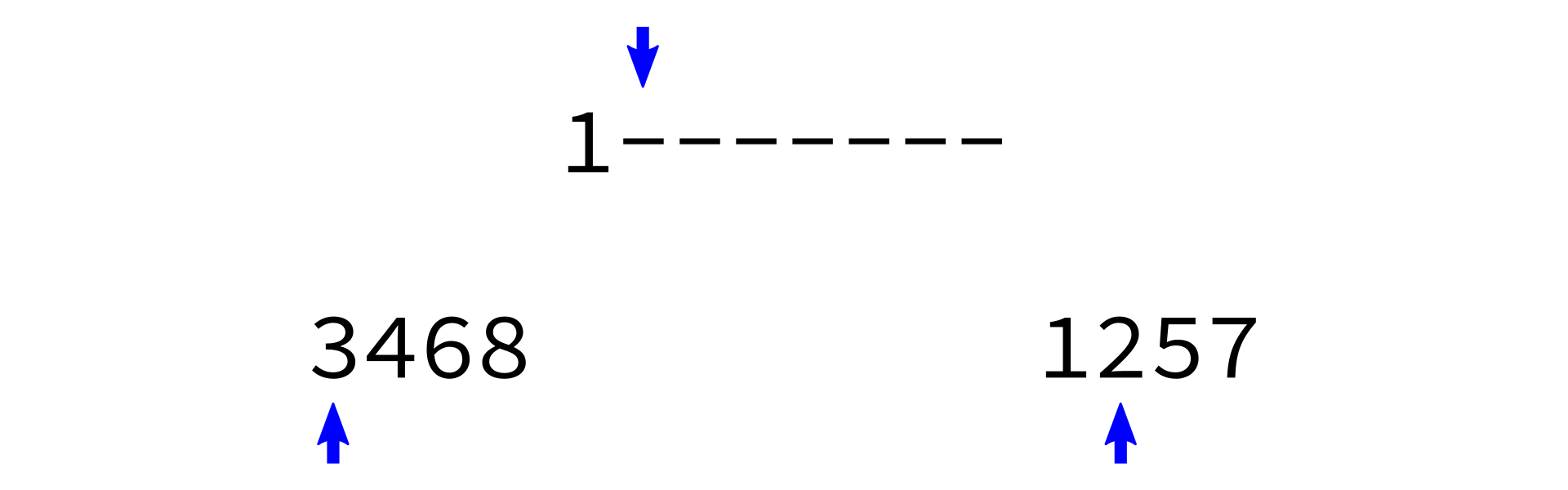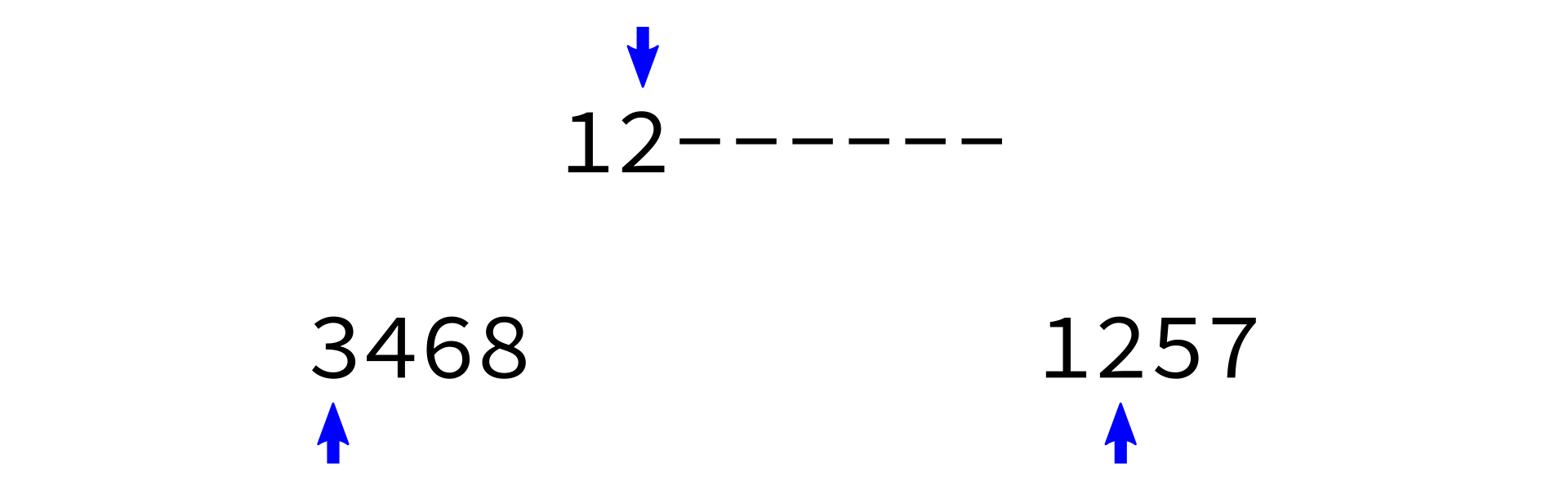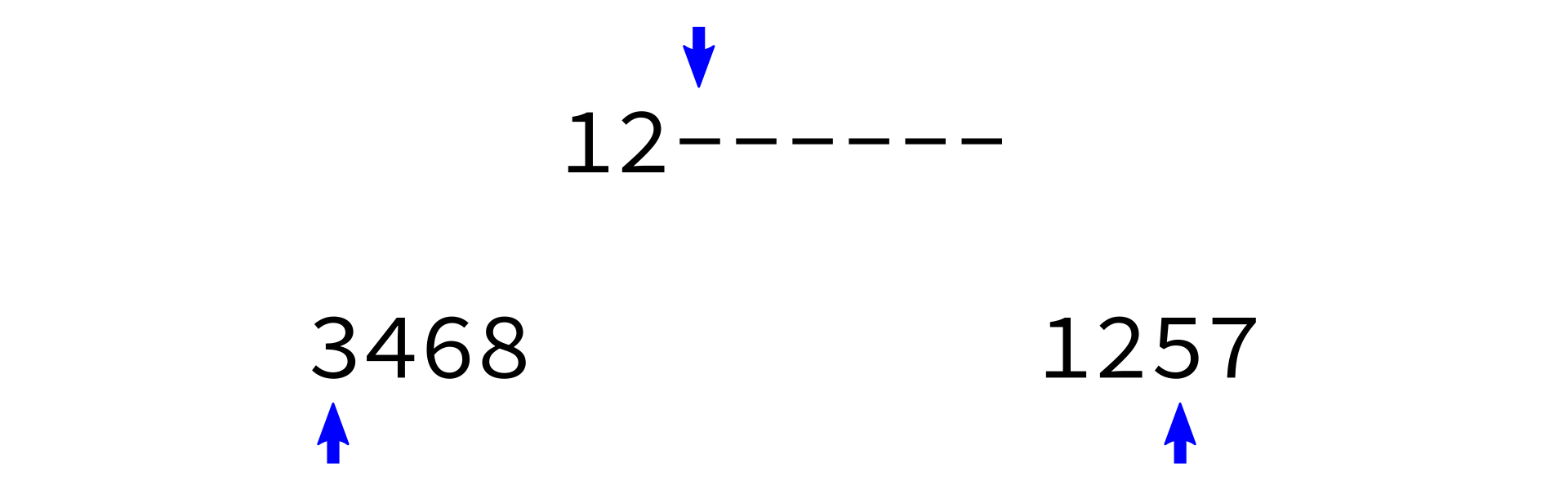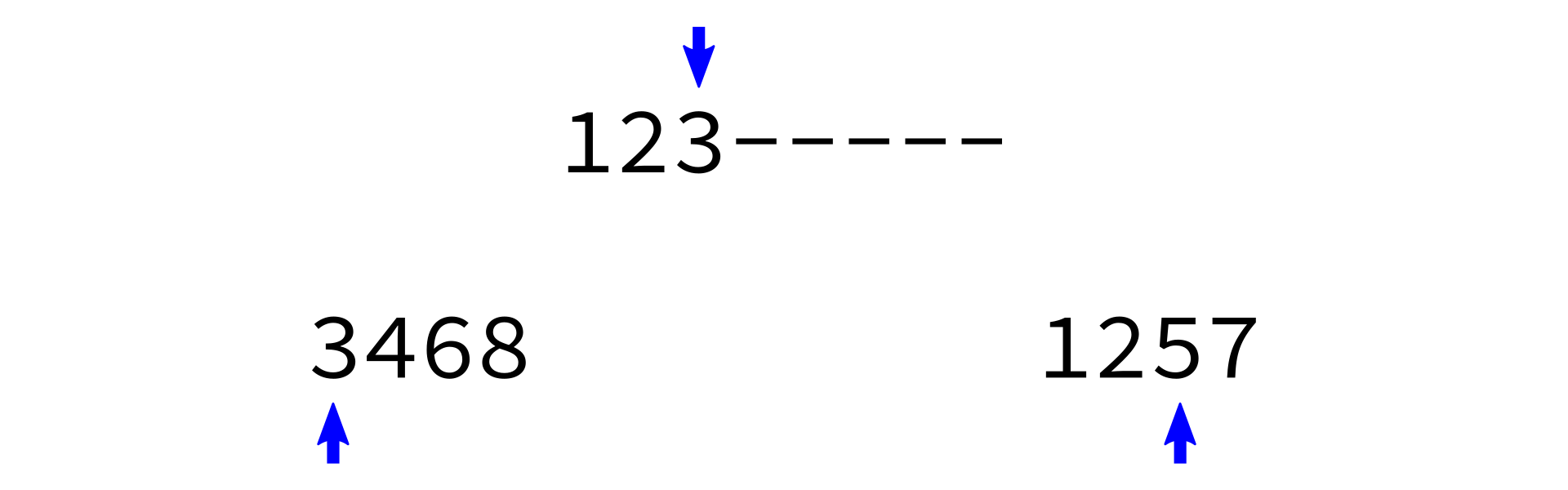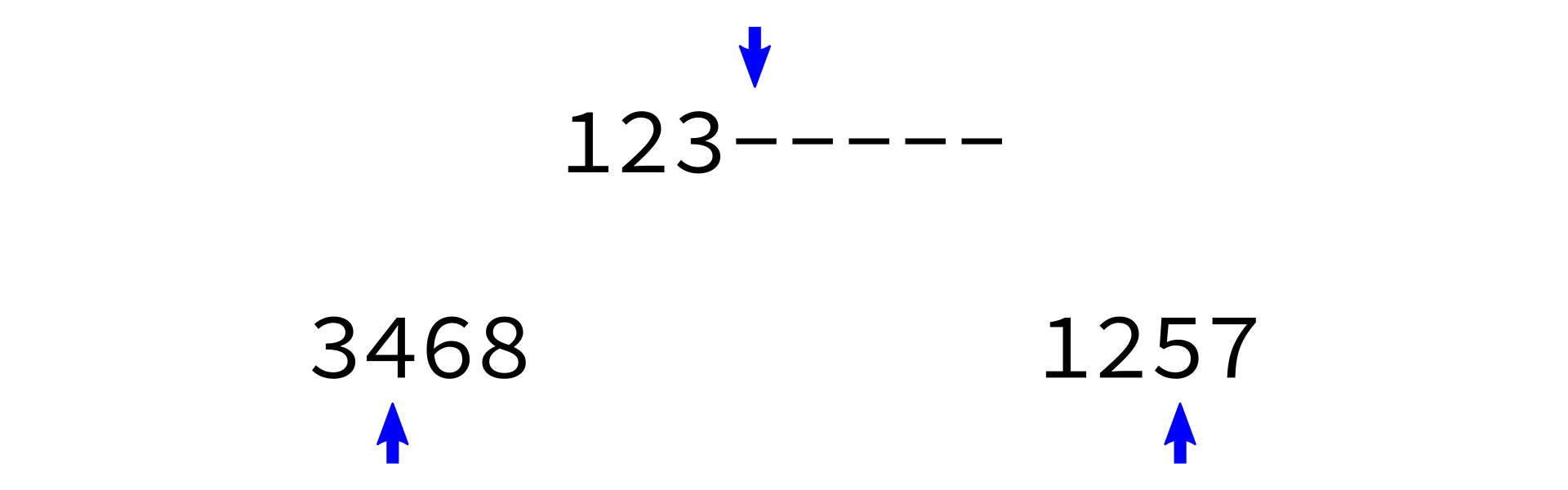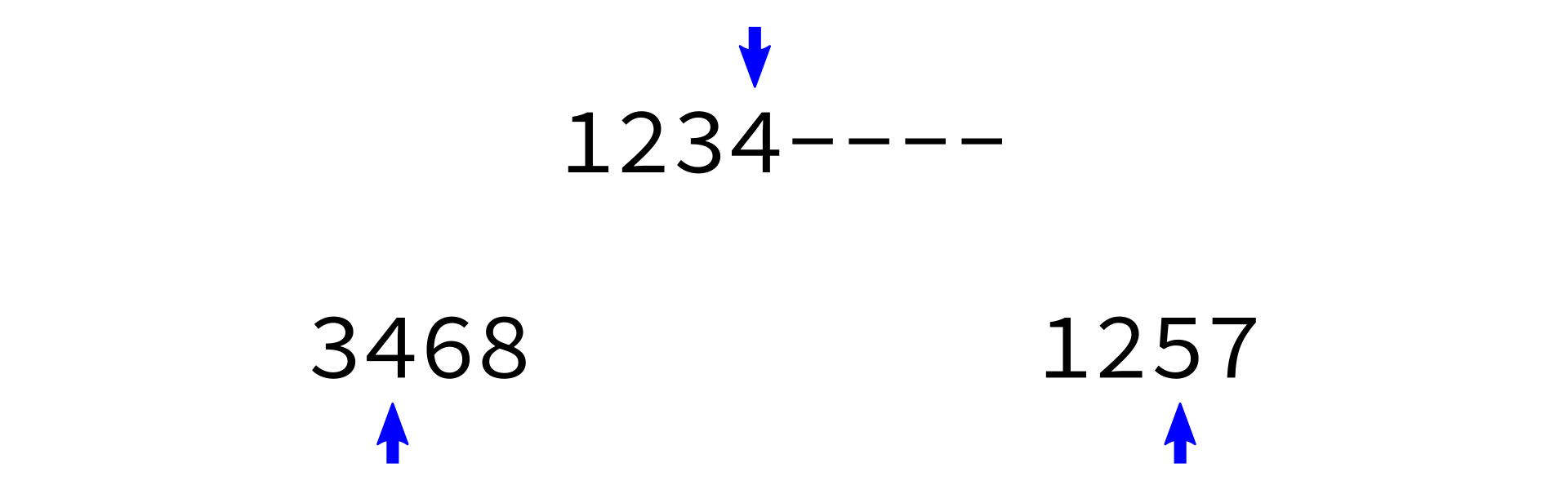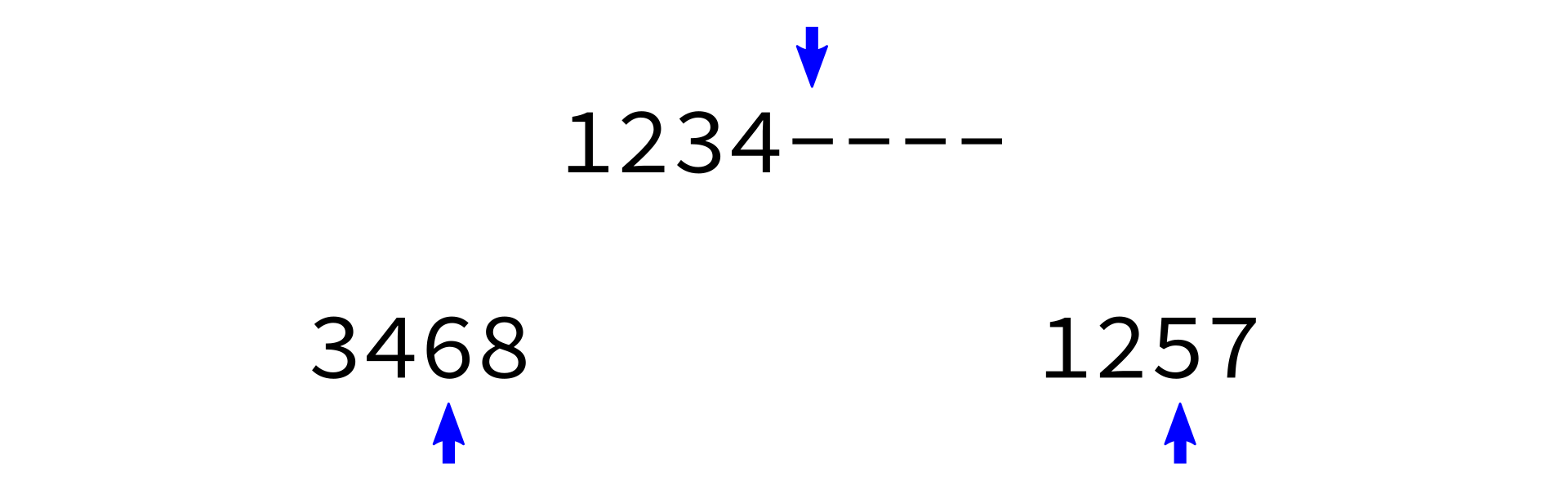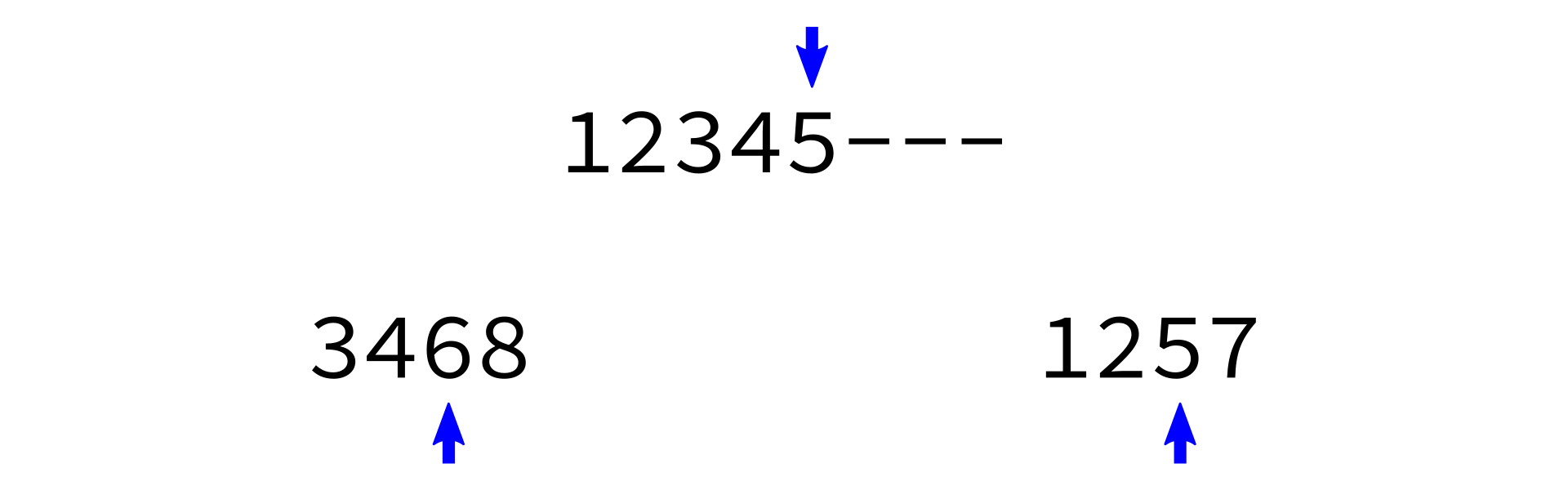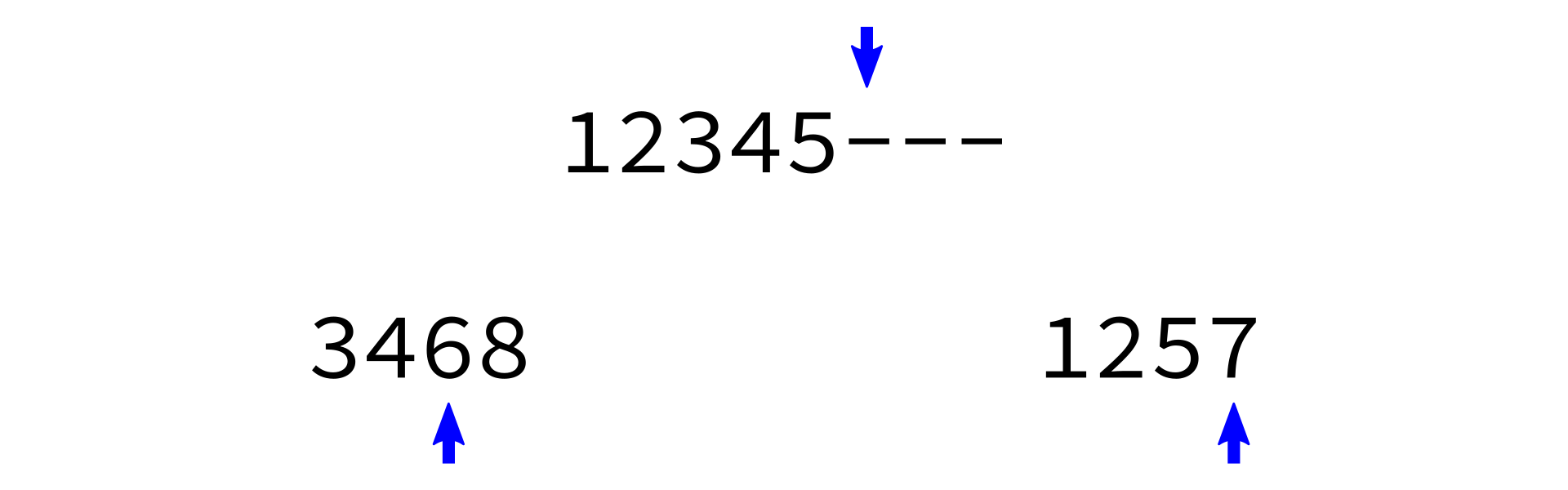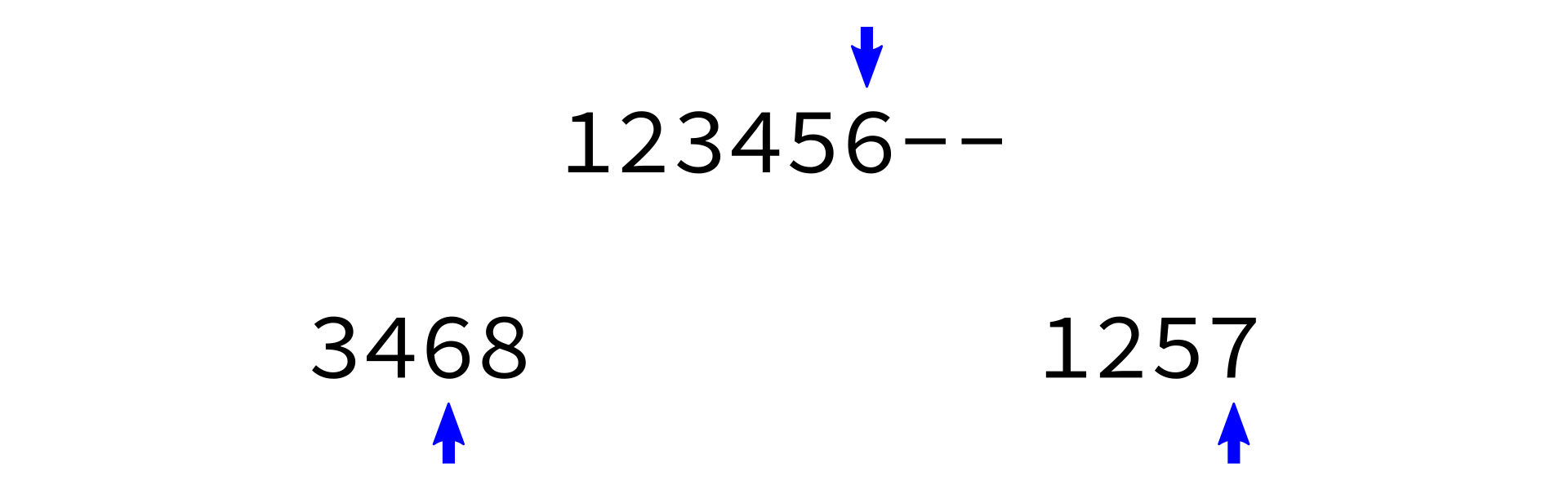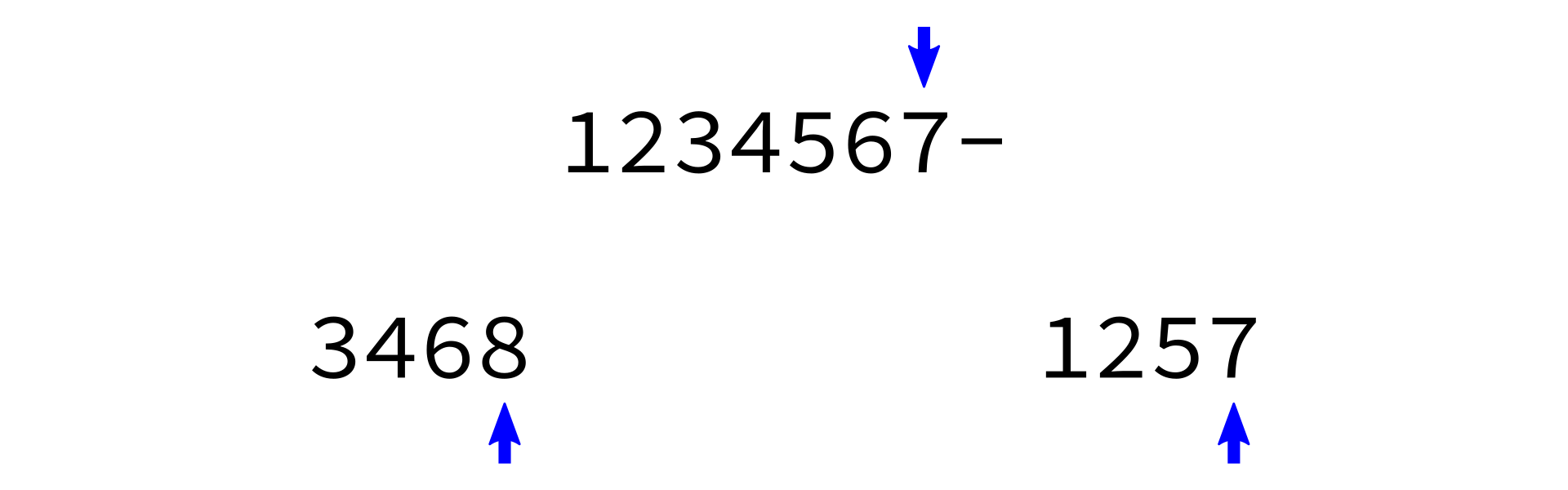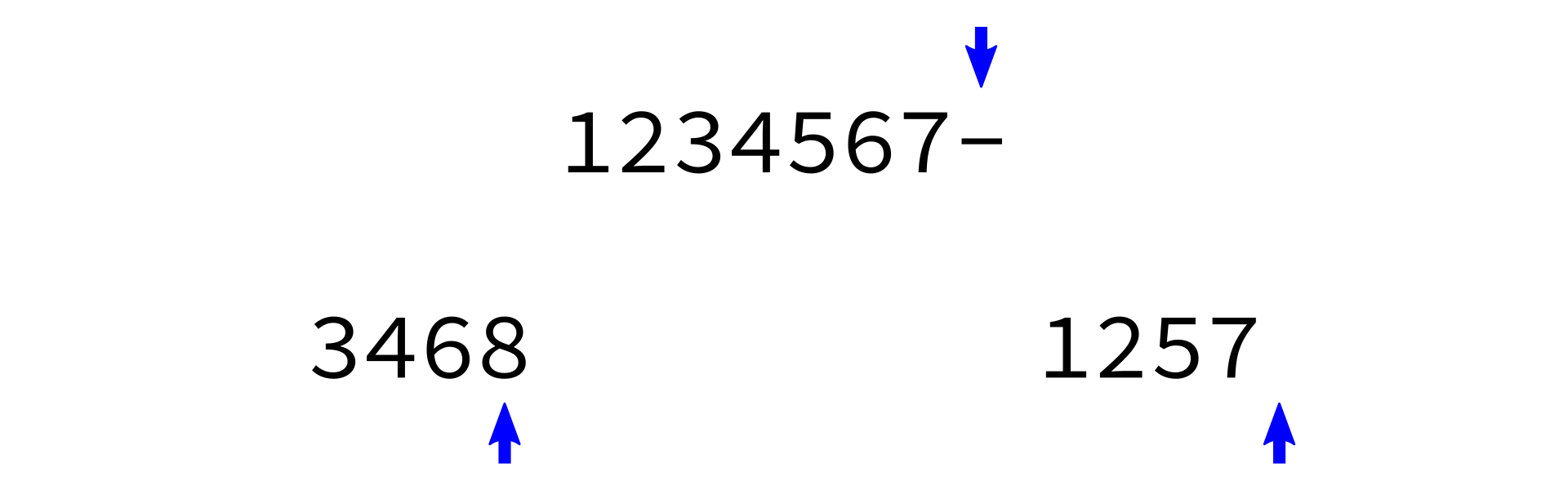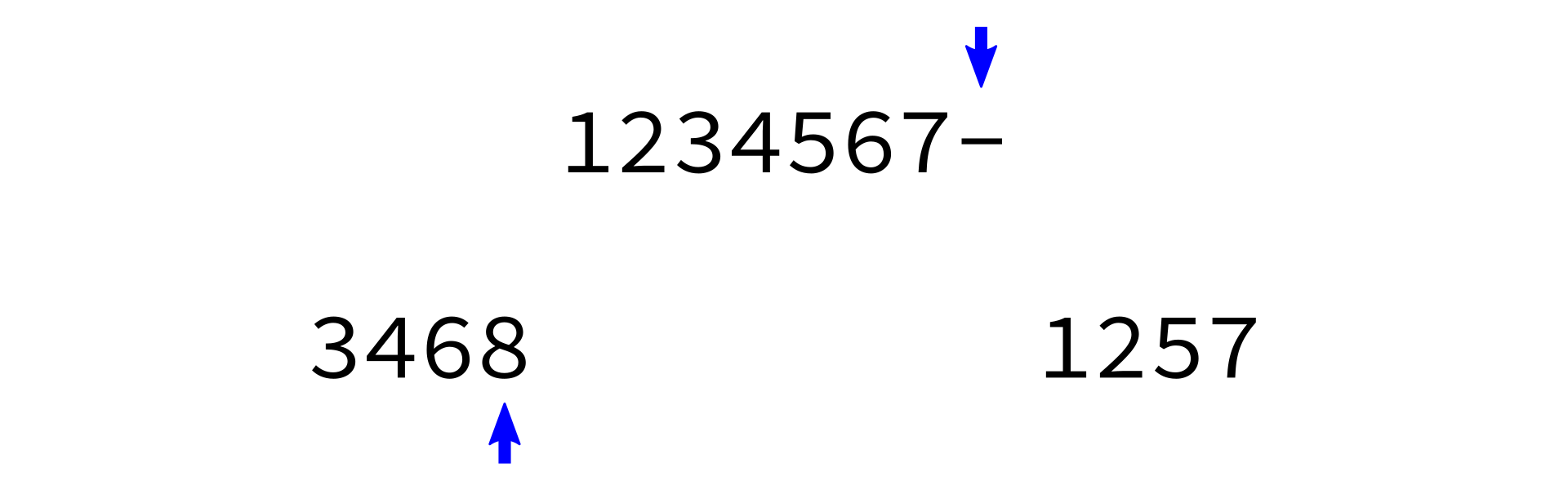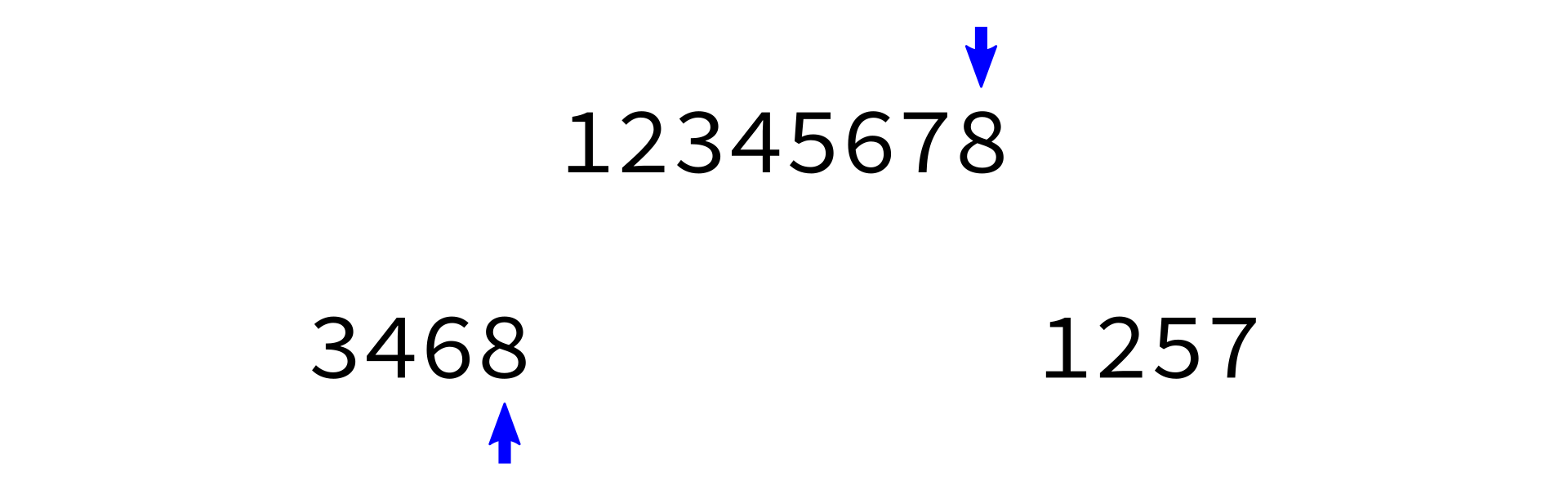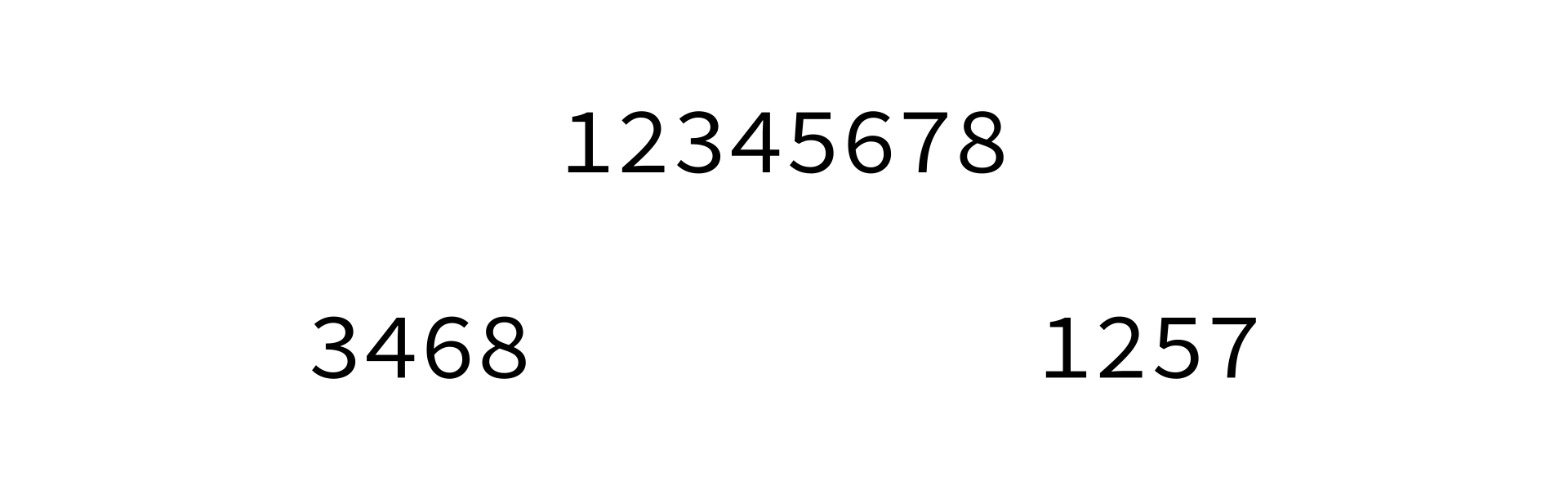## Coding time

Let's implement mergesort in Python.